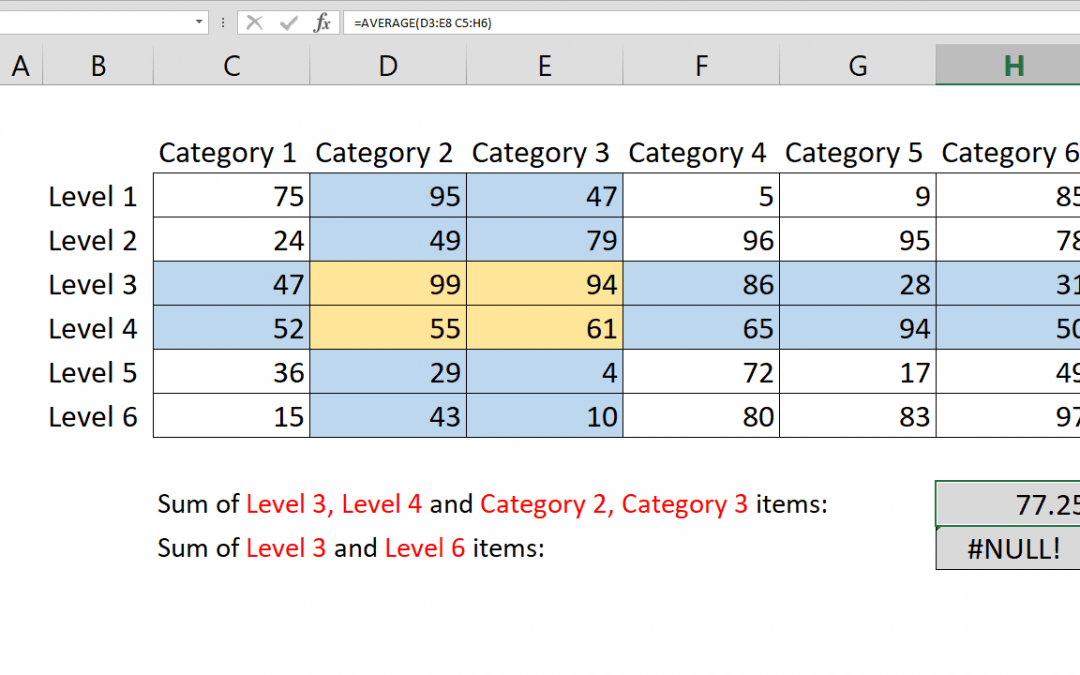Select Page

## Windows Character Set (1252)

Windows Character Set is a one byte character encoding system derived from Latin alphabet. The character set shares same characters up to first 128 character with ASCII character encoding. Excel’s the CHAR function uses this characters set for Windows versions....

## MAC OS Character Set

Mac OS Character Set is based on one byte character encoding system, Mac-Roman. The character set shares same characters up to first 128 character with ASCII character encoding. Excel’s the CHAR function uses this characters set for Mac versions. Code...## How to use double quotes in Excel formulas

Excel uses double quote character to identify text, you need to a different approach. This article shows you How to use double quotes in Excel formulas in two ways: with and without a function. Syntax =”””” =CHAR(34) How There are 2 ways to use...## How to calculate AVERAGE with intersecting tables

This article shows how to calculate average with intersecting tables using AVERAGE function and intersect operator, the space character (” “). Syntax =AVERAGE(first range second range) Steps Type =AVERAGE( function Select or type the first range reference...## Count values with Excel intersect operator

Excel intersect operation is handled by the space character (” “). You can intersect multiple rages by space in range supporting formulas. This article shows how to COUNT intersecting ranges with COUNT function and Excel intersect operator. Syntax...## How to calculate SUM with intersecting tables

This article shows how to calculate sum with intersecting tables using SUM function and intersect operator, the space character (” “). Syntax =SUM(first range second range) Steps Type =SUM( function Select or type the first range reference that includes...## How to lookup across two tables with VLOOKUP and IF functions

How to lookup across two tables by a condition is a combination of the VLOOKUP and the IF functions. The IF function handles which table and VLOOKUP handles what to search for. Syntax =VLOOKUP(lookup value, IF(condition, first table if condition is TRUE, second table...## How to calculate monthly averages

This article shows you how to calculate monthly averages from a data table containing dates and values using AVERAGEIFS and EOMONTH functions. If you only want to calculate the number of days in a month, please refer to the article: How to find number of days in month...